## 8.4.1 Feedback Motion Planning Definitions

Using the concepts from Section 8.3, we are now ready to define feedback motion planning over configuration spaces or other continuous state spaces. Recall Formulation 4.1, which defined the basic motion planning problem in terms of configuration space. The differences in the current setting are that there is no initial condition, and the requirement of a solution path is replaced by a solution vector field. The formulation here can be considered as a continuous-time adaptation to Formulation 8.1.

Formulation 8..2 (Feedback Motion Planning)
1. A state space,, which is a smooth manifold. The state space will most often be, as defined in Section 4.3.1.8.9
2. For each state,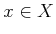, an action space,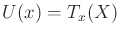. The zero velocity,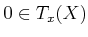, is designated as the termination action,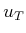. Using this model, the robot is capable of selecting its velocity at any state.8.10
3. An unbounded time interval,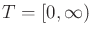.
4. A state transition (differential) equation,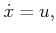(8.38)

which is expressed using a coordinate neighborhood and yields the velocity,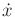, directly assigned by the action. The velocity produced byis(which means stop'').
5. A goal set,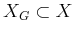.

A feedback plan,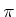, for Formulation 8.2 is defined as a function, which produces an action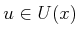for each. A feedback plan can equivalently be considered as a vector field onbecause eachspecifies a velocity vector (specifies zero velocity). Since the initial state is not fixed, it becomes slightly more complicated to define what it means for a plan to be a solution to the problem. Let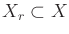denote the set of all states from which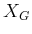is reachable. More precisely, a state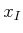belongs to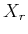if and only if a continuous path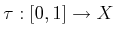exists for which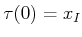and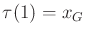for some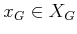. This means that a solution path exists fromfor the open-loop'' motion planning problem, which was considered in Chapter 4.

Subsections
Steven M LaValle 2020-08-14